Interpreting Slope

Learning Objectives

• Differentiate between a positive relationship and a negative relationshipFigure 1. This skier speeds down the slope in an Olympic race. What’s your guess as to the steepness, or slope, of this ski hill?

What the Slope Means

The concept of slope is very useful in economics, because it measures the relationship between two variables. A positive slope means that two variables are positively related—that is, when x increases, so does y, and when x decreases, y also decreases. Graphically, a positive slope means that as a line on the line graph moves from left to right, the line rises. We will learn in other sections that “price” and “quantity supplied” have a positive relationship; that is, firms will supply more when the price is higher.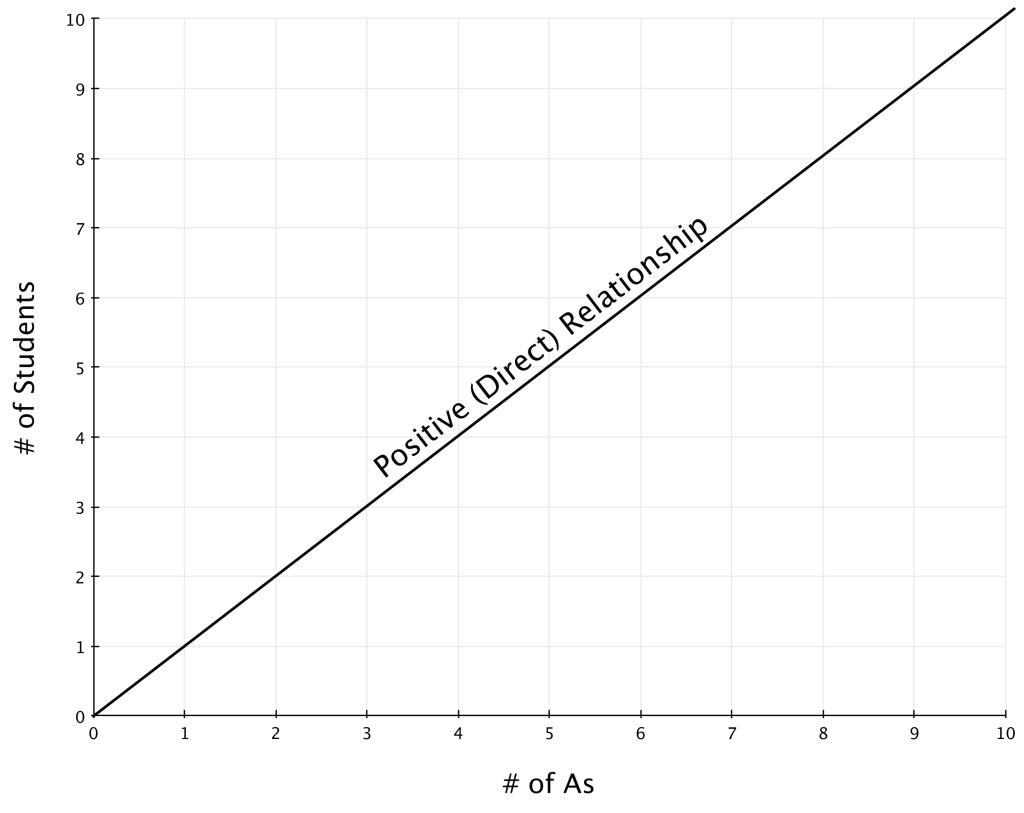Figure 1. Positive Slope.

A negative slope means that two variables are negatively related; that is, when x increases, y decreases, and when x decreases, y increases. Graphically, a negative slope means that as the line on the line graph moves from left to right, the line falls. We will learn that “price” and “quantity demanded” have a negative relationship; that is, consumers will purchase less when the price is higher.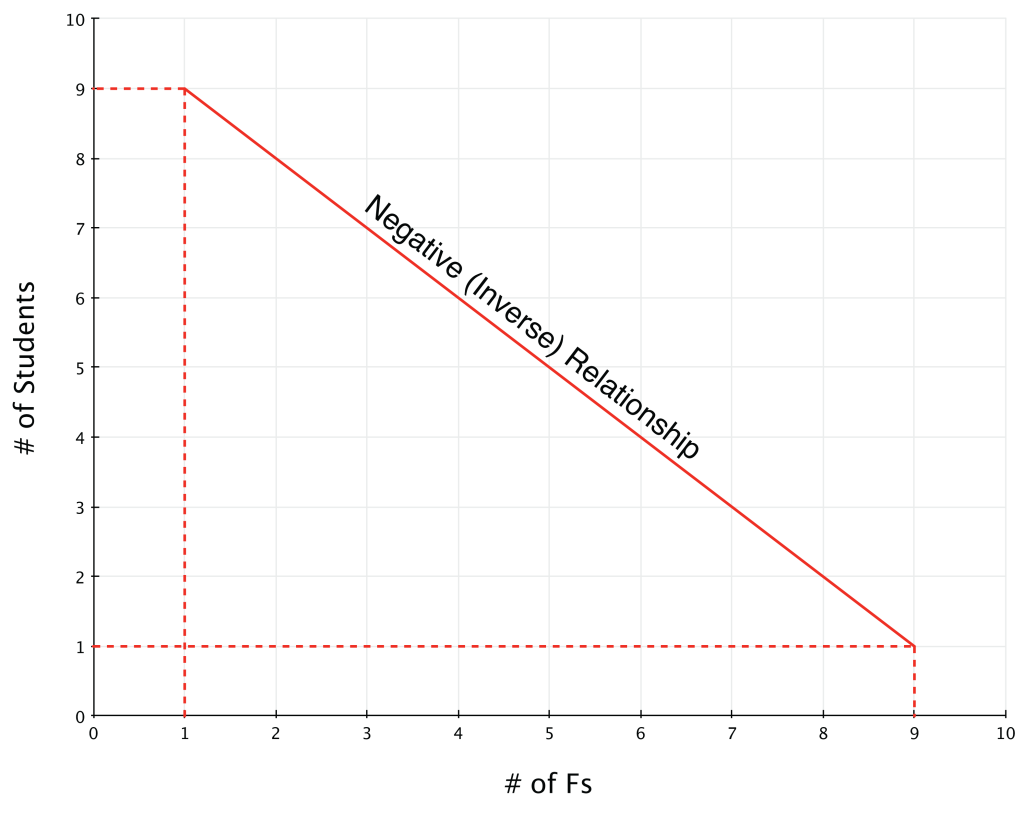Figure 2. Negative slope.

A slope of zero means that y is constant no matter the value of x. Graphically, the line is flat; the rise over run is zero.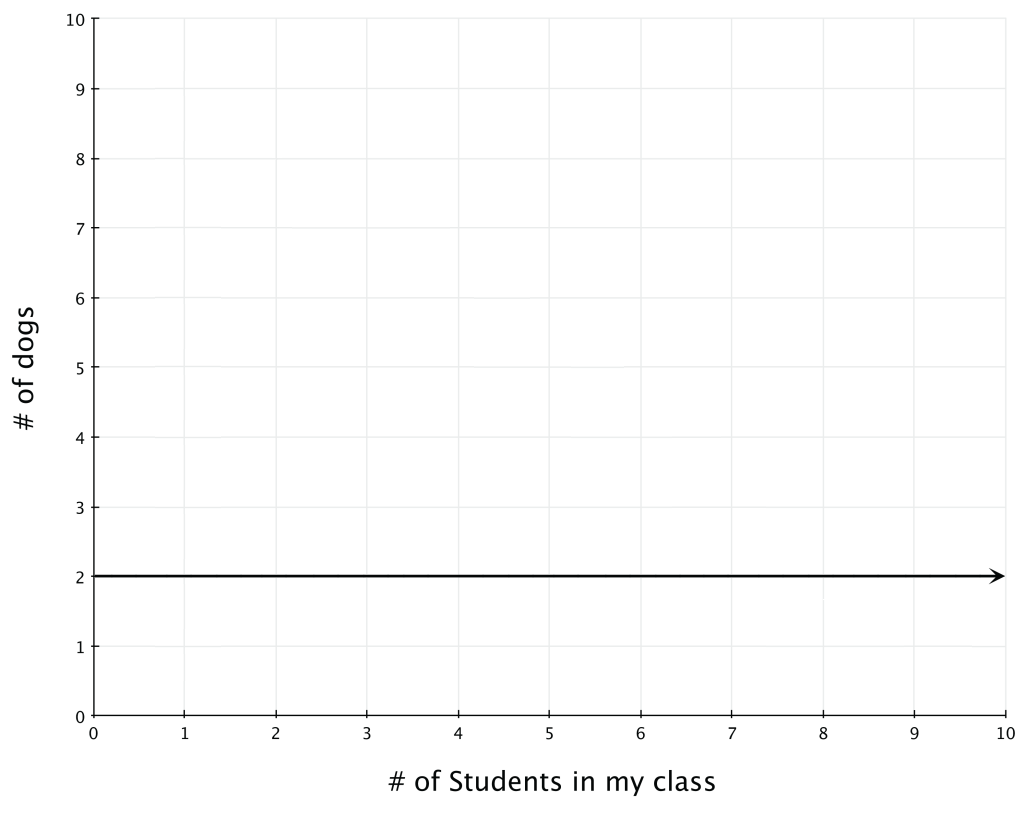Figure 3. Slope of Zero

The unemployment-rate graph in Figure 4, below, illustrates a common pattern of many line graphs: some segments where the slope is positive, other segments where the slope is negative, and still other segments where the slope is close to zero.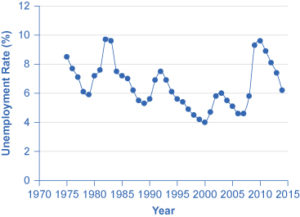Figure 4. U.S. Unemployment Rate, 1975–2014.

Calculating Slope

The slope of a straight line between two points can be calculated in numerical terms. To calculate slope, begin by designating one point as the “starting point” and the other point as the “end point” and then calculating the rise over run between these two points.

Try It

Use the graph to find the slope of the line.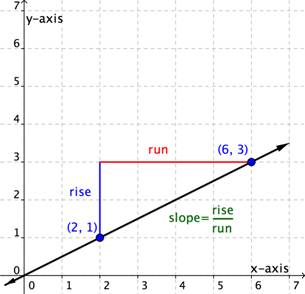Figure 5.

Start from a point on the line, such as $(2,1)$ and move vertically until in line with another point on the line, such as $(6,3)$. The rise is 2 units. It is positive as you moved up.

Next, move horizontally to the point $(6,3)$. Count the number of units. The run is 4 units. It is positive as you moved to the right.

Then solve using the formula:

$\displaystyle \text{Slope }=\frac{\text{rise}}{\text{run}}$

so

$\displaystyle \text{Slope}=\frac{2}{4}=\frac{1}{2}$

Try It

These next questions allow you to get as much practice as you need, as you can click the link at the top of the first question (“Try another version of these questions”) to get a new set of questions. Practice until you feel comfortable doing the questions and then move on.

Graphs of economic relationships are not always straight lines. In this course, you will often see nonlinear (curved) lines, like Figure 6, which shows the relationship between quantity of output being produced and the cost of producing that output. As the quantity of output increases, the total cost increases at a faster rate. Table 1 shows the data behind this graph.

 Table 1: Total Cost Curve Quantity of Output (Q) Total Cost (TC) 1 $1 2$4 3 $9 “Point A” 4$16 “Point B” 5 $25 6$36 7 $49 8$64 9 $81 10$100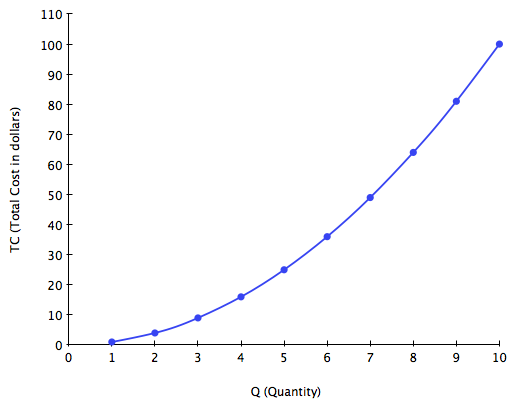Figure 6. In this example, the total cost of production increase at a faster rate when the quantity of output increases.

We can interpret nonlinear relationships similarly to the way we interpret linear relationships. Their slopes can be positive or negative. We can calculate the slopes similarly also, looking at the rise over the run of a segment of a curve.

As an example, consider the slope of the total cost curve, above, between points A & B.  Going from point A to point B, the rise is the change in total cost (i.e. the variable on the vertical axis):

$25 –$16 = $9 Similarly, the run is the change in quantity (i.e. the variable on the horizontal axis): 5 – 4 = 1 Thus, the slope of a straight line between these two points would be 9/1 = 9. In other words, as we increase the quantity of output produced by one unit, the total cost of production increases by$9.

Try It

Suppose the slope of a line were to increase. Graphically, that means it would get steeper. Suppose the slope of a line were to decrease. Then it would get flatter. These conditions are true whether or not the slope was positive or negative to begin with. A lower positive slope means a flatter upward tilt to the curve, which you can see in Figure 6 at low levels of output. A higher positive slope means a steeper upward tilt to the curve, which you can see at higher output levels.

A negative slope that is larger in absolute value (that is, more negative) means a steeper downward tilt to the line. A slope of zero is a horizontal line. A vertical line has an infinite slope.

Suppose a line has a larger intercept. Graphically, that means it would shift out (or up) from the old origin, parallel to the old line. This is shown in Figure 7, below, as the shift from the line labeled Y to the line labeled Y1. If a line has a smaller intercept, it would shift in (or down), parallel to the old line.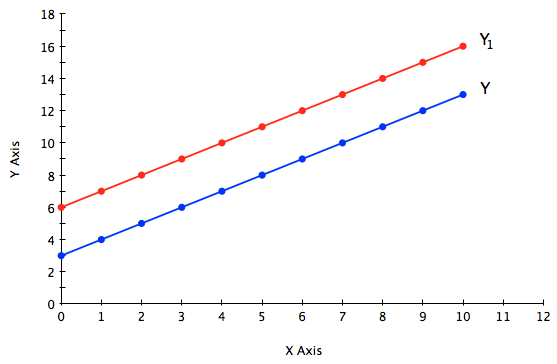Figure 7. A larger y-intercept shifts the entire graph to cross the y-axis at a higher point.

Glossary

negative slope:
indicates that two variables are negatively related; when one variable increases, the other decreases, and when one variable decreases, the other increases
positive slope:
indicates that two variables are positively related; when one variable increases, so does the other, and when one variable decreases, the other also decreases
slope:
the change in the vertical axis divided by the change in the horizontal axis
slope of zero:
indicates that there is no relationship between two variables; when one variable changes, the other does not change